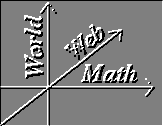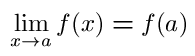#Continuity

Pre-requisites: Continuous Functions., Limits.

Just like before, a scalar valued function of several variables is continuous at a point if and only if

• it is defined at that point and if the limit of the function is the point.
That is if f is the function in question and a is the point
• f(a) exists.
• f(x) must have a limit as x approaches a.
•Definition: A curve is simply a continuous map from an interval in R to R2 or R3. A curve sigma(t): I -> R3 is graphed by plotting all the points in its image, sigma(I) = { sigma(t): t in I }.

Here are some examples of curves:

• Lines. Here the interval I is taken to be all of R, and the curve is l(t) = OP + t v. If you want a ray instead of a line, make I into [0,infinity), and if you want a line segment let I be a closed interval [a,b].

• A circle. I = [0,2 pi] and sigma(t) = ( cos t, sin t ). A circle is a closed curve because sigma(0) = sigma(2 pi). cos t and sin t are called the component functions of the curve. A subarc of the circle, I = [a,b] with 0 < a < b < 2 pi would not be closed because the ends of the subarc, sigma(a) and sigma(b), would not be equal.

• A helix. sigma(t) = ( cos t, sin t, t ) and I = R.

• The graph of a continuous function f(t) can be made into a curve by setting sigma(t) = ( t, f(t) ).

Vector Calculus Index | World Web Math Main Page

watko@athena.mit.edu